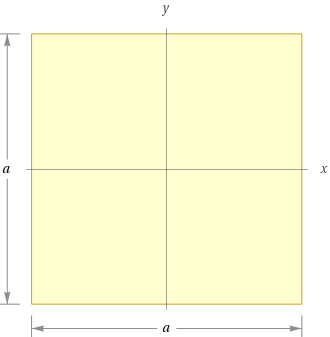Search IntMath
Close

# The Difference Between Square and Rectangle in Geometry## What is Square Geometry?

Square geometry is a type of two-dimensional shape that has four equal sides and four right angles. All of the sides are equal in length and the angles measure 90�. The opposite sides of a square are parallel and the opposite angles are equal. The area of a square is calculated by multiplying the length of one side by itself.

The diagonals of a square are equal in length and they bisect each other at right angles. The diagonals also divide the square into four congruent triangles. All of the sides of a square meet at one point, which is known as the center or vertex.

## What is Rectangle Geometry?

Rectangle geometry is a type of two-dimensional shape that has four sides and four angles. The angles of a rectangle measure 90�. The opposite sides of a rectangle are parallel and the opposite angles are equal. The area of a rectangle is calculated by multiplying the length of one side by the width of the other.

The diagonals of a rectangle are not equal in length and they do not bisect each other at right angles. The diagonals of a rectangle also divide the shape into four congruent triangles. All of the sides of a rectangle meet at two points, which are known as the vertices.

## How Are Square and Rectangle Geometry Different?

The main difference between square and rectangle geometry is that the sides of a square are equal in length while the sides of a rectangle are not. Additionally, the diagonals of a square are equal in length and bisect each other at right angles, while the diagonals of a rectangle are not equal in length and do not bisect each other at right angles.

The area of a square is calculated by multiplying the length of one side by itself, while the area of a rectangle is calculated by multiplying the length of one side by the width of the other. Lastly, all of the sides of a square meet at one point, while all of the sides of a rectangle meet at two points.

## Practice Problems

1. What is the area of a rectangle with a length of 10 cm and a width of 5 cm?

Answer: The area of the rectangle is 50 cm2.

2. What is the area of a square with sides that measure 6 cm?

Answer: The area of the square is 36 cm2.

3. What type of shape has four equal sides and four right angles?

4. What type of shape has four sides and four angles, but the opposite sides are not equal in length?

5. What is the length of the diagonals of a square with sides that measure 5 cm?

Answer: The length of the diagonals of the square is 7.07 cm.

## Conclusion

Square and rectangle geometry have many similarities and differences. Both shapes have four sides and four angles, but the sides of a square are equal in length while the sides of a rectangle are not. The area of a square is calculated by multiplying the length of one side by itself, while the area of a rectangle is calculated by multiplying the length of one side by the width of the other. Lastly, the diagonals of a square are equal in length and bisect each other at right angles, while the diagonals of a rectangle are not equal in length and do not bisect each other at right angles.

## FAQ

### What is the difference between a square and a rectangle?

The main difference between a square and a rectangle is that a square has all sides of equal length, whereas a rectangle has two pairs of sides with different lengths. A square also has equal angles of 90 degrees, while a rectangle has angles that can vary.

### What shape has four equal sides?

A shape with four equal sides is a square.

## Problem SolverThis tool combines the power of mathematical computation engine that excels at solving mathematical formulas with the power of GPT large language models to parse and generate natural language. This creates math problem solver thats more accurate than ChatGPT, more flexible than a calculator, and faster answers than a human tutor. Learn More.